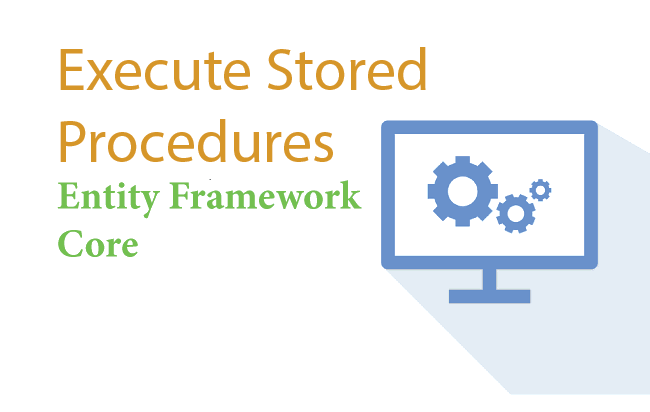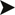# Execute SQL Stored Procedures using FromSqlRaw() & ExecuteSqlRawAsync() methods in Entity Framework CoreSQL Stored Procedures can be easily executed using the FromSqlRaw() & ExecuteCommand() methods in Entity Framework Core. This tutorial will cover them in details.#### This tutorial is a part of Entity Framework Core series.

On my last tutorial called Execute Raw SQL Queries using FromSqlRaw() method in Entity Framework Core I created the entities and Database Context. I will continue to use them in this tutorial to.
Stored Procedure

Below is the SQL Stored Procedure that returns all Students that have a particular name and standard. The name & standard values are provided through the name & standard input parameters of the Stored Procedure.

``````CREATE PROCEDURE [dbo].[sp_GetStudents]
@Name       VARCHAR(50),
@Standard   INT
AS
BEGIN
SELECT * FROM Student Where Name=@Name AND Standard=@Standard
END``````

## Entity Framework Core Execute Stored Procedure with “FromSqlRaw()” method

The above stored procedure has 2 input parameters which have to be provided when using the FromSqlRaw() method. Therefore we have to use SqlParameter array to sets these parameters.

The SqlParameter class resides inside Microsoft.Data.SqlClient namespace.

The below code executes the stored procedure.

``````var param = new SqlParameter[] {
new SqlParameter() {
ParameterName = "@Name",
SqlDbType =  System.Data.SqlDbType.VarChar,
Size = 100,
Direction = System.Data.ParameterDirection.Input,
Value = "Tony"
},
new SqlParameter() {
ParameterName = "@Standard",
SqlDbType =  System.Data.SqlDbType.Int,
Direction = System.Data.ParameterDirection.Input,
Value = 10
}};
List<Student> studentList = context.Student.FromSqlRaw("[dbo].[sp_GetStudents] @Name, @Standard", param).ToList();``````

### Stored Procedures that return Mulitple Record Sets

Remember that the .FromSqlRaw() method gets only one record set from a stored procedure. If the Stored procedure returns multiple record sets then use the ExecuteReader() method.

The below procedure returns 2 record sets – one from Student table and other from StudentAddress table.

``````CREATE PROCEDURE [dbo].[sp_MultiRecordSets]
@IdStu   INT,
AS
BEGIN
SELECT * FROM Student Where Id=@IdStu
END``````

This is how to use ExecuteReader() method to get the values from both the record sets.

```var param = new SqlParameter[] {
new SqlParameter() {
ParameterName = "@IdStu",
SqlDbType =  System.Data.SqlDbType.Int,
Direction = System.Data.ParameterDirection.Input,
Value = 5
},
new SqlParameter() {
SqlDbType =  System.Data.SqlDbType.Int,
Direction = System.Data.ParameterDirection.Input,
Value = 10
}};

var context = new SchoolContext();
using (var cnn = context.Database.GetDbConnection())
{
var cmm = cnn.CreateCommand();
cmm.CommandType = System.Data.CommandType.StoredProcedure;
cmm.CommandText = "[dbo].[sp_MultiRecordSets]";
cmm.Connection = cnn;
cnn.Open();

{
// name from student table
}
reader.NextResult(); //move the next record set
{
// city from student address table
}
}
```

Note : NextResult() moves to the next record set.

What are the 3 most important topics of Entity Framework Core ?

## Execute Stored Procedure using ExecuteSqlRawAsync()

The Entity Framework Core ExecuteSqlRawAsync() method is used to execute Stored Procedures and Raw SQL in asynchronous manner. But unlike FromSqlRaw() method, it returns the number of affected rows. Note that there is also another variant called ExecuteSqlRaw() which is of synchronous type.

``var rowsAffected = await context.Database.ExecuteSqlRawAsync("Update Students set Name = 'Donald Trump' where Id = 5");``

### Working with output parameters of Stored Procedure using ExecuteSqlRawAsync()

Here we have added a new Stored Procedure with one output parameter called @TotalStudents which returns the total students in the table.

```CREATE PROCEDURE [dbo].[sp_GetStudentsNew]
@Name               VARCHAR(50),
@Standard           INT,
@TotalStudents      INT            OUTPUT
AS
BEGIN
SELECT * FROM Student Where Name=@Name AND Standard=@Standard
SET @TotalStudents= (SELECT COUNT(*) FROM Student)
END
```

To execute this Stored Procedure in EF Core we will not have to provide an SqlParameter with output direction.

```new SqlParameter() {
ParameterName = "@TotalStudents",
SqlDbType =  System.Data.SqlDbType.Int,
Direction = System.Data.ParameterDirection.Output, // direction output
}
```

Once the stored procedure is executed we can get the value returned by the output parameter like this:

``int totalStudents = Convert.ToInt32(Convert.ToString(param.Value));``

Note : Parameter starts with index 0. Here there are total 3 parameters and the last one is the output parameter.

The complete code is:

```var context = new SchoolContext();

var param = new SqlParameter[] {
new SqlParameter() {
ParameterName = "@Name",
SqlDbType =  System.Data.SqlDbType.VarChar,
Size = 100,
Direction = System.Data.ParameterDirection.Input,
Value = "Tony"
},
new SqlParameter() {
ParameterName = "@Standard",
SqlDbType =  System.Data.SqlDbType.Int,
Direction = System.Data.ParameterDirection.Input,
Value = 10
},
new SqlParameter() {
ParameterName = "@TotalStudents",
SqlDbType =  System.Data.SqlDbType.Int,
Direction = System.Data.ParameterDirection.Output,
}};
int affectedRows = await context.Database.ExecuteSqlRawAsync("[dbo].[sp_GetStudentsNew] @Name, @Standard, @TotalStudents out", param);
int totalStudents = Convert.ToInt32(param.Value);
```